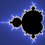# NMTC Problem 3a

The Fibonacci Sequence is defined by $F_0 = 1$,$F_1 =1$ and $F_n=F_{n-1}+F_{n-2}$. Prove that $7{F_{n+2}^3}-{F_n^3}-{F_{n+1}^3}$ is divisible by $F_{n+3}$.

This a part of my set NMTC 2nd Level (Junior) held in 2014.Note by Siddharth G
6 years, 8 months ago

This discussion board is a place to discuss our Daily Challenges and the math and science related to those challenges. Explanations are more than just a solution — they should explain the steps and thinking strategies that you used to obtain the solution. Comments should further the discussion of math and science.

When posting on Brilliant:

• Use the emojis to react to an explanation, whether you're congratulating a job well done , or just really confused .
• Ask specific questions about the challenge or the steps in somebody's explanation. Well-posed questions can add a lot to the discussion, but posting "I don't understand!" doesn't help anyone.
• Try to contribute something new to the discussion, whether it is an extension, generalization or other idea related to the challenge.

MarkdownAppears as
*italics* or _italics_ italics
**bold** or __bold__ bold
- bulleted- list
• bulleted
• list
1. numbered2. list
1. numbered
2. list
Note: you must add a full line of space before and after lists for them to show up correctly
paragraph 1paragraph 2

paragraph 1

paragraph 2

[example link](https://brilliant.org)example link
> This is a quote
This is a quote
    # I indented these lines
# 4 spaces, and now they show
# up as a code block.

print "hello world"
# I indented these lines
# 4 spaces, and now they show
# up as a code block.

print "hello world"
MathAppears as
Remember to wrap math in $$ ... $$ or $ ... $ to ensure proper formatting.
2 \times 3 $2 \times 3$
2^{34} $2^{34}$
a_{i-1} $a_{i-1}$
\frac{2}{3} $\frac{2}{3}$
\sqrt{2} $\sqrt{2}$
\sum_{i=1}^3 $\sum_{i=1}^3$
\sin \theta $\sin \theta$
\boxed{123} $\boxed{123}$

Sort by:

Let us take $F_{n}=x$ and $F_{n+1}=y \Rightarrow\ F_{n+2}=x+y \Rightarrow\ F_{n+3}=x+2y.$Now,let us expand the given expression:$: 7F_{n+2}^{3}-F_{n}^{3}-F_{n+1}^{3}$ in terms of $x$ and $y$.We get$: 7(x+y)^{3}-x^{3}-y^{3}$.Simplifying that,we get$: 6x^{3}+6y^{3}+21xy(x+y).$Taking $(x+y)$ common we get$: (x+y)(6x^{2}-6xy+6y^{2}+21xy) =(x+y)(6x^{2}+15xy+6y^{2}) =(x+y)(x+2y)(6x+3y).$But,$(2y+x)=F_{n+3}.$hence proved:):).

- 6 years, 8 months ago

- 6 years, 8 months ago

Absolutely right. This is wht I tried, but the $X,Y$ substitution, Mindblowing :D

- 6 years, 8 months ago

thanx!!

- 6 years, 8 months ago

Perfect! I didn't go for x,y and faced problems in factorizing. PS: Small Typo at the end.

- 6 years, 8 months ago

thanx!!

- 6 years, 8 months ago

yes thanku!!

- 6 years, 8 months ago

The substitution certainly helped made it easier to manipulate.

Staff - 6 years, 8 months ago

Yes, however, can we do this with induction?

- 6 years, 8 months ago

Possibly, but I don't see why that would work, nor do I see a way to start.

There is very little here to motivate a solution by induction. Knowing divisibility by $F_n$ doesn't tell you anything about divisibility by $F_{n+1}$.

Staff - 6 years, 8 months ago

How did you do?

- 6 years, 8 months ago

Not well, 1b 5a, b 6b and half of the 8th were good. I left 1a half done. How was your paper?

- 6 years, 8 months ago

Sigh, atleast you had one full question to your credit. I got the first one fully , messed up second one (after getting half of it), third one wasn't salubrious, (I tried both, got to almost the answers), 4th I didnt do, 5a I didnt know, 5 b I got it, 6- I wrote Yes and No alternatingly :P, 7th I almost got it but lost it due to calculation error (You wont believe I put 40 cubed= 16000 :( )..8th I am not sure of it's accuracy....

Now , you clearly know I sucked more than you did. :(

- 6 years, 8 months ago

Same problem with wet 3a. BTW are you sure that 6a is 'Yes'?

- 6 years, 8 months ago

Oh Nono, I didnt understand it properly but gave some stupid explanation and put Yes. WBU? How did you do that question?

- 6 years, 8 months ago

6a. Forthe transformation, we needed $\Delta B=+2$ and $\Delta O=0$. However with the allowed changes, these conditions were contradicting each other.

- 6 years, 8 months ago

Well, as I said...I wasnt even considering that question right :P. How was NTSE? (Range of marks..?)

- 6 years, 8 months ago

Terrible! Expecting 114/140; expected cutoff~123

- 6 years, 8 months ago

Oh, I am sorry for bringing that up. How did you predict the cutoff so soon? (Institute?)...And, Could you tell me the number of seats for general cat. in Delhi.

- 6 years, 8 months ago

Not really, mostly from the marks of competitive peers. The no. of seats was 60 last year, however, I think they are going to increase it. FIITJEE predicts it to be near 119 due to this.

- 6 years, 8 months ago

Oh I see. Be optimistic though :)

- 6 years, 8 months ago

Nah, just moving on. Are you giving RMO?

- 6 years, 8 months ago

Yes I am. But NMTC has almost you know, shattered the vital mathematical force in me :3

- 6 years, 8 months ago

- 6 years, 8 months ago

Good. xD. It ough'to be that good lest you wouldn't qualify in TN. Expecting 130+, let's see.(the results)

- 6 years, 8 months ago